# 正则 reg ——文本搜索、替换

### 语法：修饰符、方括号、元字符

• /正则表达式主体/修饰符(可选)
• 修饰符——执行区分大小写和全局匹配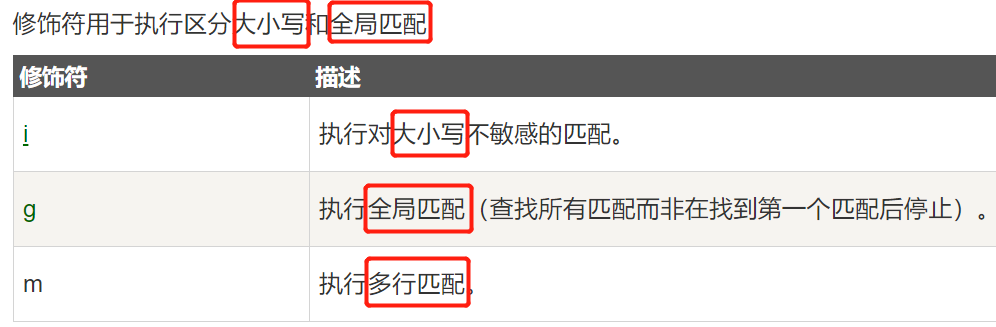• 方括号——用于查找某个范围内的字符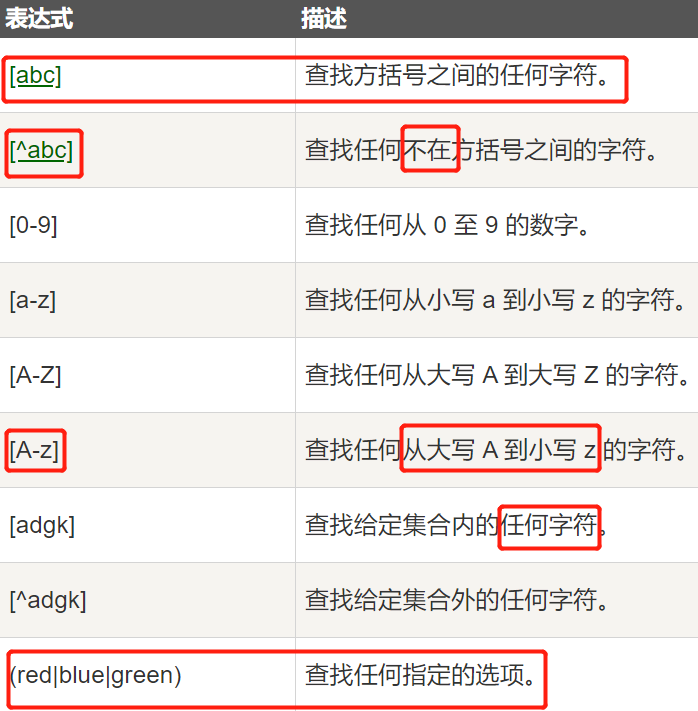• 元字符——是拥有特殊含义的字符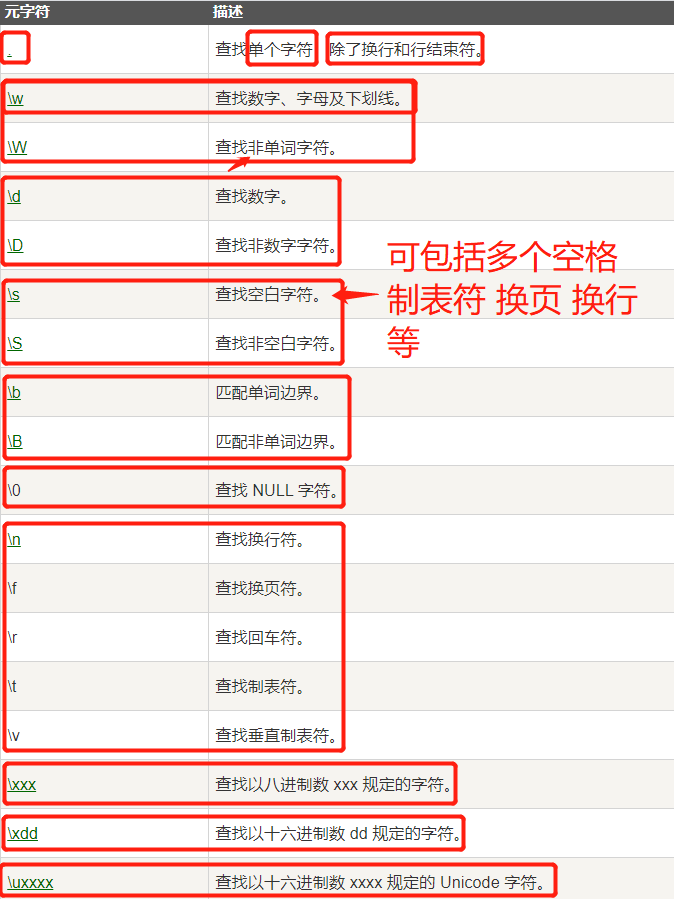• 量词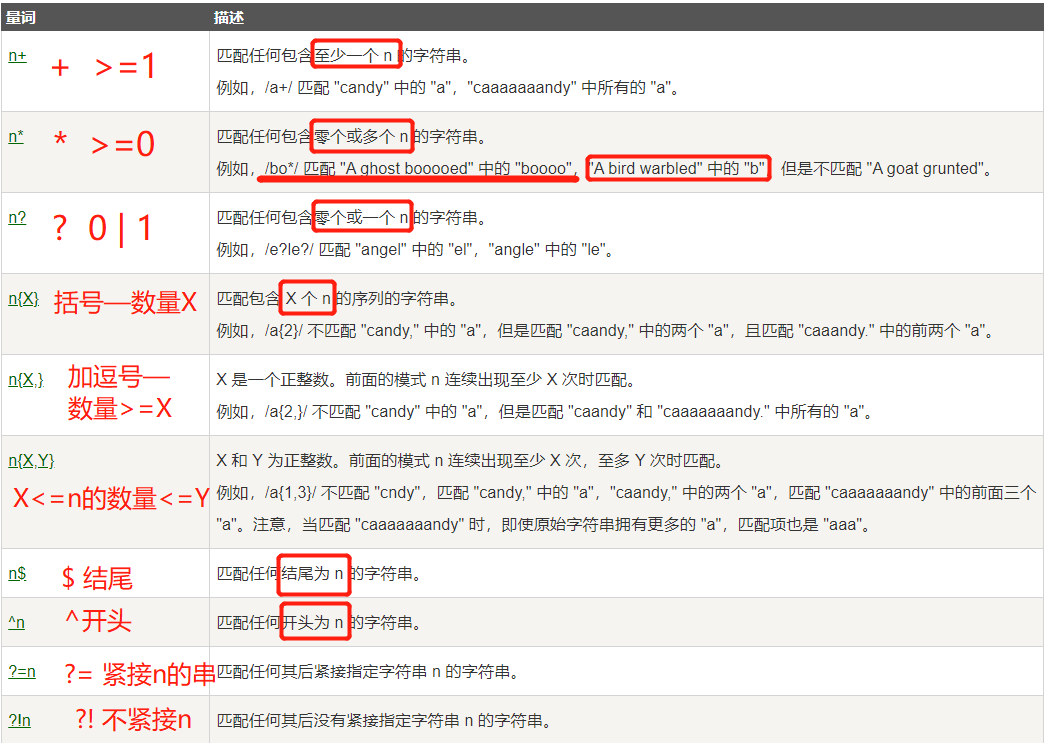• RegExp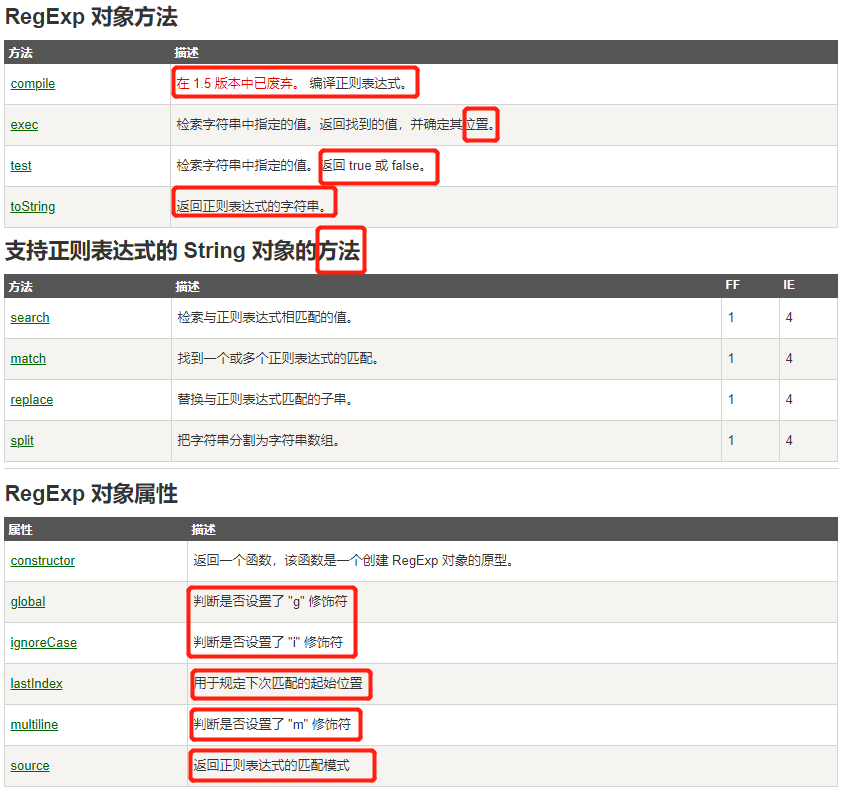### 搭配search() 方法

• 检索字符串中指定的子字符串，或检索与正则表达式相匹配的子字符串
• 返回子串的起始位置

### 搭配replace() 方法

• 用一些字符替换另一些字符，或替换一个与正则表达式匹配的子串
• \s+ 匹配任意多个空白字符
• \f -> 匹配一个换页
• \n -> 匹配一个换行符
• \r -> 匹配一个回车符
• \t -> 匹配一个制表符
• \v -> 匹配一个垂直制表符

# 字符串 string 操作 分割、连接

### 查找

• string.includes(searchvalue, start)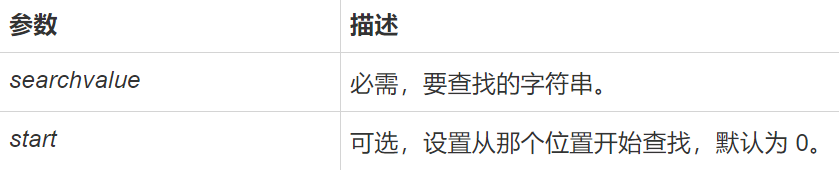### 划分

• split() 括号内为划分的依据 按照其划分
• substr()
• substring()

### 转为字符串 toString()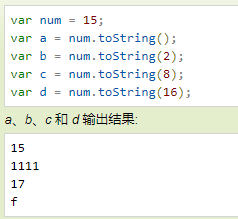### JS中的 大小、长度问题

• 集合 哈希表的长度 用 size
• 用对象的长度是否为0 判断 对象是否为空

### 疑问：res.push([…temp]) 与 res.push(temp) ??

• temp为数组
• res.push([…temp]) //[[1,2,3]]
• res.push(temp) //[[]]

### ! 判断还有元素 用while(obj.length)

• 不能while(obj) ，要用length

# 数组 array

• arr.shift() 删除并返回数组的第一个元素 【树也可以使用】
• arr.unshift() 头插入
• arr.push 尾插入数组添加对象元素
• arr.pop()
• arr.splice()
• arr.slice()
• arr.indexOf(data,[index]) 寻找指定元素的下标，可以从指定下标找起
• arr.sort 排序 arr.sort(function(a,b){a-b});
• arr.concat()
• arr.split()
• arr.join() 用指定字符连接字符串
• arr.forEach 循环遍历数组
• arr.map 重构数组，不会创建新对象
• arr.filter 过滤数组，不会创建新数组
• arr.some 检查数组中是否有指定条件 判断大于 小于
• arr.includes 判断数组中是否包含某值
• arr.valueOf() 返回数组对象的原始值
• arr.find(function(data){}) 寻找符合条件的值第一个
• arr.toString() 转换字符串
• arr.entries() 方法返回一个数组的迭代对象，该对象包含数组的键值对 (key/value)
在这里插入代码片


# 深拷贝

### JSON解析

	let t = JSON.stringify(matrix)
t = JSON.parse(t)


### 数组扩展[…arr]

• 可以深复制一维数组，二维复制后仍有使用问题
let t = [...matrix]    //展开一维数组


# ES6

• 不存在变量提升
• 变量生命前为暂时性死区
• 常用于for循环中

• 声明时，必须赋值
• 无法对值修改
• 不存在变量提升
• 变量生命前为暂时性死区

### 变量解构 （数组、对象）

【用法】

• 基础解构

let [foo, [[bar], baz]] = [1, [, 3]];
foo // 1
bar // 2
baz // 3

let [ , , third] = ["foo", "bar", "baz"];
third // "baz"

let [x, , y] = [1, 2, 3];
x // 1
y // 3

let [head, ...tail] = [1, 2, 3, 4];
tail // [2, 3, 4]

let [x, y, ...z] = ['a'];
x // "a"
y // undefined
z // []

• 对象解构 （对象的属性没有次序变量必须与属性同名，才能取到正确的值）

let { bar, foo } = { foo: 'aaa', bar: 'bbb' };
foo // "aaa"
bar // "bbb"

let { baz } = { foo: 'aaa', bar: 'bbb' };
baz // undefined

• 不完全解构

let [x, y] = [1, 2, 3];
x // 1
y // 2

let [a, [b], d] = [1, [2, 3], 4];
a // 1
b // 2
d // 4


【作用】

1. 交换变量值 [x, y] = [y, x];

2. 从函数返回多个值

// 返回一个数组
function example() {
return [1, 2, 3];
}
let [a, b, c] = example();

// 返回一个对象
function example() {
return {
foo: 1,
bar: 2
};
}
let { foo, bar } = example();

3. 遍历 Map 结构

const map = new Map();
map.set('first', 'hello');
map.set('second', 'world');

for (let [key, value] of map) {
console.log(key + " is " + value);
}
// first is hello
// second is world


只想获取键名[key]/键值[,value]:

// 获取键名
for (let [key] of map) {
// ...
}
// 获取键值
for (let [,value] of map) {
// ...
}

4. 函数参数的定义

5. 提取 JSON 数据

6. 函数参数的默认值

7. 输入模块的指定方法

const { SourceMapConsumer, SourceNode } = require("source-map");


# JS Math

• 文档
【对象属性】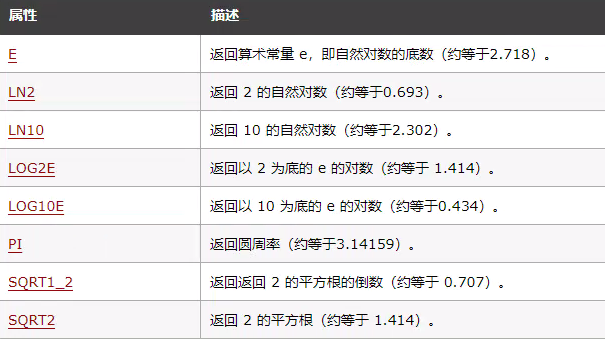Math.E //2.718281828459045
Math.LN2 //0.6931471805599453
Math.SQRT1_2 //0.7071067811865476


【方法属性】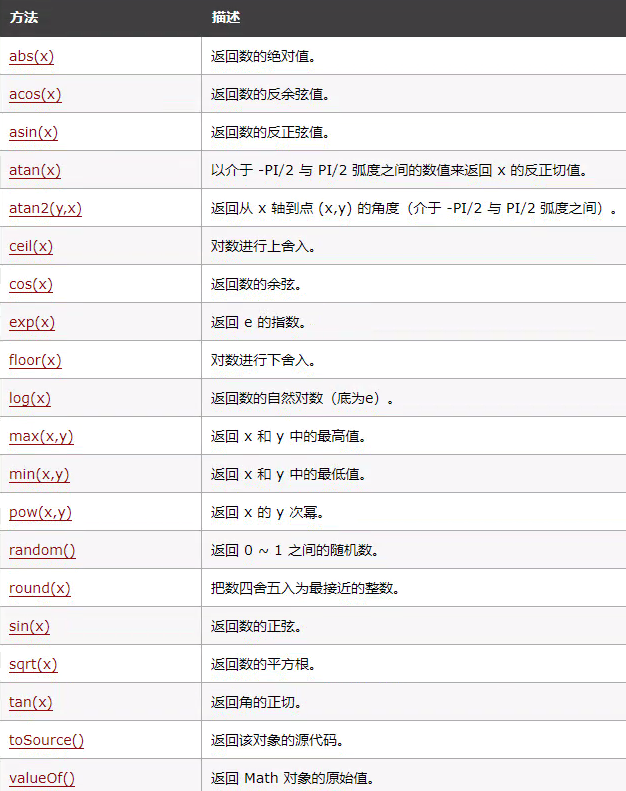• abs(x) 返回数的绝对值。
Math.abs(7.25-10)  //2.75

• round(x) 把数四舍五入为最接近的整数。
Math.round(0.60)  // 1
Math.round(-4.40)  //-4
Math.round(-4.6)  //-5

• ceil(x) 对数进行上舍入。
Math.ceil(0.60)  // 1
Math.ceil(-5.9)  //-5

• floor(x) 对数进行下舍入。
• random() 返回 0 ~ 1 之间的随机数
• exp(x) 返回 e 的指数。
• sqrt(x) 返回数的平方根。
• valueOf() 返回 Math 对象的原始值。
• max(x,y) 返回 x 和 y 中的最高值。min(x,y)类似
• pow(x,y) 返回 x 的 y 次幂。

# JS 位操作 参考笔记

• 1.&（与）
都是1才是1

• 2.|（或）
有一个是1就是1

• 3.^（异或）
不同就是1

• 4.~(非)
0变1，1变0 (JavaScript仅支持32位整型数)

• 5.<<(有符号左移)
有符号左移会将32位二进制数的所有位向左移动指定位数
num<<n相当于num乘以2的次方

var num = 5; // 二进制111
num << 3; // 二进制111000，十进制40

• 6.>>(有符号右移)
num>>n相当于num除以2的次方

(64).toString(2)  //"1000000"
(64>>3).toString(2)  //"1000",十进制8


【注意】有符号左移与右移不会影响符号位。

• 7.>>>(无符号右移)

var num = -64;       //11111111111111111111111111000000
(num >>> 5).toString(2); //"111111111111111111111111110",十进制134217726


【Tips】>> << ~有时候会用来取整

• 一般我们取整会用以下这些方式其中一种：

parseInt(1.5) //1
Math.round(1.5) //2
Math.floor(1.5) //1
Math.ceil(1.5) //2

• 但是有时候我们想追求更高的性能，我们会考虑用位运算来取整：

1.5>>0 //1
1.5<<0 //1
~~1.5 //1


### JS函数的简洁写法

const dfs = (参数) => {
函数内容
}


### 移动到不等元素位置：

while (nums[l] === nums[++l]);


### sort()

• 调用时没有参数，将按字母顺序对数组中的元素进行排序

• 如果想按照其他标准进行排序，就需要提供比较函数，该函数要比较两个值，然后返回一个用于说明这两个值的相对顺序的数字

• 例子：

nums.sort((a, b) => a - b) // 升序排列


### 判断为NaN

isNaN(res)


### === 与 == 区别

• == 代表相同，类型不同，也有可能相等，需根据以下规则进行类型转换在比较：
• 如果一个是null，一个是undefined，那么相等
• 如果一个是字符串，一个是数值，把字符串转换成数值之后再进行比较
• ===代表严格相同，类型不同，就一定不相等

### !==和 !=的区别

• != 会转换成相同类型 进行比较
• !== 除了比对值还比对类型

### 反转

• 方法一：字符反转
• 数字变字符：let str = x+'' 或者 .toString()
• 方法二：数字除法和取余数
• 一行：分割为数据，反转函数，再拼接
x.toString().split('').reverse().join('')


### 创建一个m*n的二维数组

• 数组的初始值为0
   var res = new Array(m).fill(0).map(() => new Array(n).fill(0));

10-11259
07-313045
05-10101
03-26406
05-021571
09-16297
01-2293
04-182562
12-31483
11-067798
08-1570
02-12979
11-23213
03-31266
08-23570
08-145103
03-271万+
10-092万+
07-21209

### “相关推荐”对你有帮助么？

•非常没帮助
•没帮助
•一般
•有帮助
•非常有帮助被折叠的  条评论 为什么被折叠?到【灌水乐园】发言¥2 ¥4 ¥6 ¥10 ¥20余额支付 (余额：-- )扫码支付获取中扫码支付点击重新获取扫码支付1.余额是钱包充值的虚拟货币，按照1:1的比例进行支付金额的抵扣。
2.余额无法直接购买下载，可以购买VIP、C币套餐、付费专栏及课程。余额充值